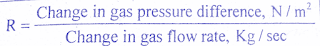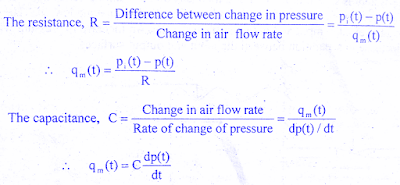# Transfer Function of Pneumatic System

0
161

## Transfer Function of Pneumatic System:

#### (adsbygoogle = window.adsbygoogle || []).push({}); The two basic elements of the pneumatic system are resistance and capacitance. The restrictions in the pipes and valves offer resistance to gas flow.The gas flow resistance, R is defined as the rate of change in gas pressure difference for a change in gas flow rate. We will learn about the derivation of the transfer function of the Pneumatic system.#### The pneumatic capacitance is defined for a pressure vessel and depends on the type of expansion process involved. The capacitance of a pressure vessel may be defined as the ratio of change in gas stored for a change in gas pressure.### Transfer function of Pneumatic System derivation:

#### A simple pneumatic system is shown in the below figure and it consists of pneumatic bellows in line with the restriction. The pneumatic bellows consist of a hollow chamber with thin pneumatic walls. The sidewalls of bellows are corrugated and the input and output surface is flat. An increase in pressure within the bellows results in an increase in separation between the input and output surfaces.#### Let             Pi = Steady state value of input air pressure

pi = Increase in the pressure air source
P = Steady State value or pressure inside the bellows
p =Increase in pressure inside the bellow
Qm = Steady state value of airflow rate
qm = Area of each flat surface of the bellows
R = Resistance of the restriction
C = Capacitance of the bellows
x= Displacement of the movable surface of the bellows

#### Force opposing the motion, fo= K x(t)where K Constant representing the stiffness of bellows.At steady state the above two forces are balanced,fb = foA p(t) = K x(t)#### On equating the two equations of qm(t) we get,#### On differentiating above equation w.r.t  t , we get,#### On substituting for p(t) and dp(t)/dt , we get,#### On taking Laplace Transform with zero initial conditions, we get,#### where t = RC = Time constant of the systemThe above equation is the required transfer function of the pneumatic system.

READ HERE  Power Factor Improvement Methods